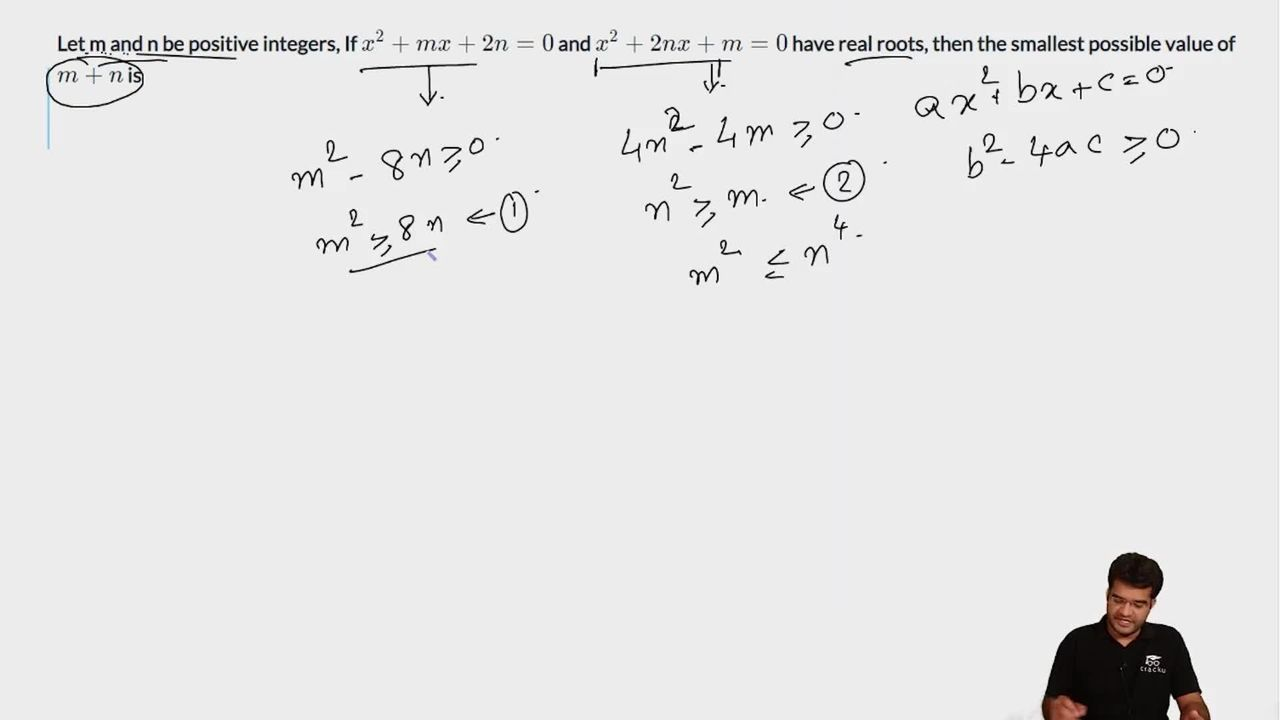Question 53

# Let m and n be positive integers, If $$x^{2}+mx+2n=0$$ and $$x^{2}+2nx+m=0$$ have real roots, then the smallest possible value of $$m+n$$ is

Solution

To have real roots the discriminant should be greater than or equal to 0.

So, $$m^2-8n\ge0\ \&\ 4n^2-4m\ge0$$

=> $$m^2\ge8n\ \&\ n^2\ge m$$

Since m,n are positive integers the value of m+n will be minimum when m=4 and n=2.

.'. m+n=6.

### View Video Solution• All Quant CAT Formulas and shortcuts PDF
• 30+ CAT previous papers with solutions PDF